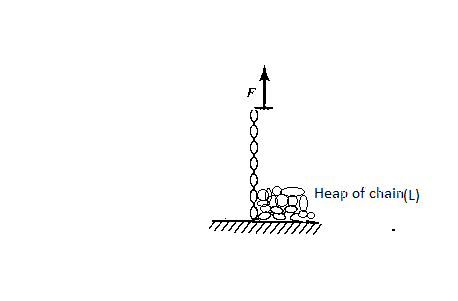# Lifting Rope.A rope of length $l$ and mass density $\lambda$ lies in a heap on a floor. You grab one end of the rope and pull upward with a non-impulsive constant force ${ F }_{ o }$. Find the time period require to completely lift off the rope.

Assume that the rope is greased.

The answer is in the form $\displaystyle{{ T=\cfrac { a }{ g } (\sqrt { \cfrac { { F }_{ o } }{ \lambda } } -\sqrt { \cfrac { { F }_{ o } }{ \lambda } -\cfrac { bgl }{ c } } })}$

Find $a+b+c$

I got this question from Deepanshu.

:D

×

Problem Loading...

Note Loading...

Set Loading...